Stability -I : Chapter 1 |

# Stability -I : Chapter 1

###### SOLUTION:

Given : L =16m , B = 15m, H = 6m, RD=0.78Volume of rectangle =(LxBxH)
=16x15x6
=1440m3

We know that :
Density = Mass /Volume
0.78 =Mass/1440m3

###### SOLUTION:

Given: Diameter = 8m,

=(8/2)m
=4m
Height =10m
Mass = 400t
RD =0.9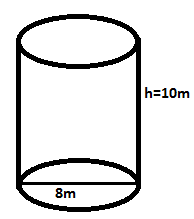We know that:
Volume of the cylindrical tank = π2h
= 3.1416 x 4 x 4 x 10
=502.656m3

We know that:
Density = (Mass/Volume)
0.9 = 400/ (Volume of the oil)

Hence, Volume of the oil = 400/0.9
=444.44m3

Depth of oil = volume/area
Area of cylinder   =( π2r)
=(3.1416 x 4 x 4)
=502656m3

We can calculate Depth of oil = (volume of oil)/ ( area of cylinder)
= (444. 44)/(50.2656 )
=8.8418 m

###### Hence, ullage  = (10 – 8.8418)  =1.1582m
1. A tank of 2400m3 volume and 12 depth, has vertical side and horizontal bottom. Find how many tonnes of oil of RD 0.7 it can hold, allowing 2% of the tank for the expansion .state the ullage of loading.

Solution :-

Given :
Volume of the tank = 2400m3
Depth of tank =12m
RD =0.7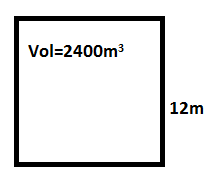According to question 2% of the volume is allowed for expansion.
As we know that

Density = Mass/ Volume
Since volume =(L x B x H)
= 2400m3( given )

So, Area = (volume / depth)
= (2400/12)
=200m2

Mass  =  ( volume x density)
Mass  =  (2400×0.7)
=1680 t

Since 2% of the volume of the tank allowed for expansion
= (2/100) x 2400
= 48m3

Volume of the oil = (volume of the tank – free space )
= ( 2400 -48)
= 2352 m3

Mass of the oil = (volume x density)
=2352 x 0.7

Depth of oil = (volume of oil /area)
= 2352/(L x B)
= (2352/ 200)
=11.76 m

###### Hence, Ullage = (12-11.76)                           = 0.24m.
1. A tank 10m deep has vertical sides .its bottom consist of triangle 12mx12mx10m. find the mass of oil (of RD0.800) to be loaded , allowing 3 % of the volume of oil loaded for expansion. State the ullage on completion of loading.

Solution:

Depth of the tank = 10m
L, B, H, of triangle = (12 x 12 x 12
RD = 0.8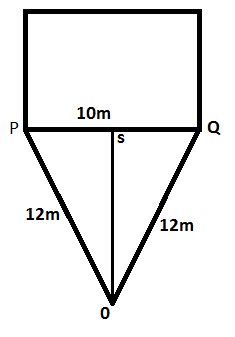Allowing, 3% of the volume of the oil loaded for expansion.
Area of the triangle = 1/2 (base x height)

In triangle PQR, RQ in the height which divide the base at same length.
So In triangle PSR

SR2 =PR2 –PS2
= (122– 52)
= (144 -25)
=119

So, SR = 10.9m
So area of the triangle = 1/2 (10×10.9)
=54.5m2

Volume of the tank = (10 x54.5)
= 545m3

Now, volume of the oil = (Total volume -free space)

let ‘V’ be the volume of the oil
545 = (V + 3/100 x V)
545 = (V + 3V/ 100)
545 = (103V /100)
V = (545 x 100) /103
V = 529.126m3

Mass of the oil = (density x volume)
= 0.8 x 529.126
=423.3 t

Depth of the oil = (volume of oil)/(area)
= (529.126 /54.5)
= 9.7087m

###### Solution :

Volume of the rectangular tank = ( L x B x H)
= (25 x 12 x 8 )

Pipe above the tank top is 0.3m

Total ullage =3.3m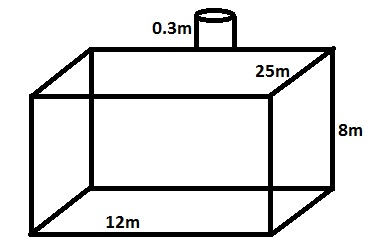Ullage inside the tank = (3.3 – 0.3)
= 3m

Depth of the SW = (8 – 3)
= 5m

Volume of the SW = (L x B x H )
= ( 25 x12 x5)
= 1500 m3

###### Given :

The volume of the rectangular tank = (L x B x H)
=(30 x16 x14)
Ullage pipe projecting above its top = 0.5m
RD of oil = 0.78
The length of the pipe line from tank to the ship = 10km
= 10000m
The diameter of the pipe = (d)
= 40cm
Hence, radius = (40 /2 )
= 20cm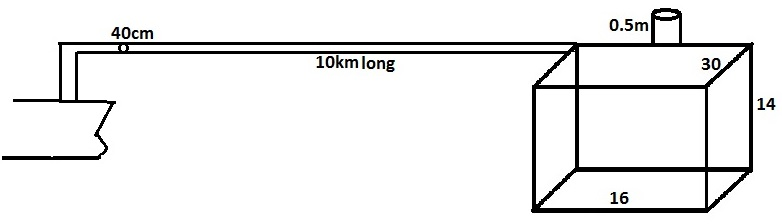Volume of the tank = (L x B x H)
= (30m x16m x14m)
=6720m3

Area = (L x B)
= (30 x 16)
= 480m2

The total ullage =0.78m

So ,ullage inside the tank = (0.78 –  0.50)
= 0.28m

If ullage inside the tank = 0.25m,The depth of oil to be loaded is
= (14 -0.28) m
=13.72m

Volume of the oil = (L x B x D)
=( 30x 16x 13.72)m
= 6585.6 m3

Mass of the oil to be load = (volume x density)
= (6585.6 x 0.78)
= 5136.768 t

Now , volume of oil in the pipe  = (πr2h)
=(3.1416 x 0.2 x 0.2 x 10000)
=1256.64m3

Depth of oil when it poured in to the tank = (volume /area)
= (1256.64 /480)
= 2.618m

Depth of the tank without the pipe line oil = (13.72 -2.618)
= 11.102m

Thus the ullage will be = (14 -11.102)
= 2.898m

###### Given :

The depth of tank = 10m
Height of the trunkway = 1m
Volume of the tank alone =8000m3
Volume of the trunkway = 500m3
The mass of the oil =5320 t
RD =0.7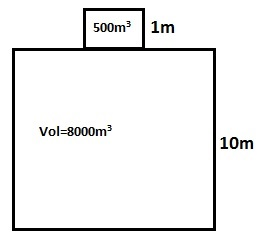We know that :
Density = (mass /volume)
Volume   = mass /density
= (5320 /0.7)
= 7600m3

Depth of oil = (volume /area)
= (7600/800)
=9.5m

Ullage insides the tank = (10 – 9.5)
=0.5m

###### Given :

The total depth of tank =21m
Volume of the tank = 20600m3
The height of the trunkway = 1m
Volume of the trunkway =  600m3
RD= 0.8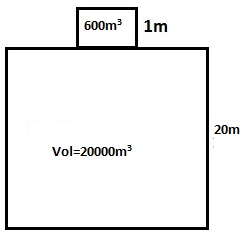The height of the tank = (21-1)
= 20m

Volume of the tank= (20600 – 600)
=20000m3

Area of tank = (20000 /20)
=10000m2

Volume of the oil = (mass /density)
=(1620 /0.8)
= 20400 m3

Hence, Volume of the oil inside the trunkway = (Total volume of the oil – volume of the tank)
= (20400 – 20000)
=400m3

Depth of the oil = (volume /area)
= (400/600)
= 0.66m

###### Given :

Depth of the tank is = 10.5m
Volume of the tank = 8200m3
Depth of the trunkway = 0.5m
Volume of the trunkway = 200m3
RD of the oil = 0.8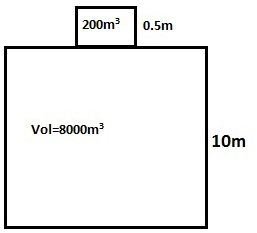According to question, 2% of the tank volume left for expansion .
Volume of the tank alone = (8200-200)
= 8000m3

Height of the tank alone = (10.5 -0.5)
= 10 m

2% of the volume of the tank left for expansion .
= (2/100 x 8200)
=164m3

Volume of the oil to be load = (8200 -164)
=8036 m3

Mass of oil to be load = ( volume x density)
= (8036 x 0.8)
= 6428.8t

Volume of the trunkway = (L x B x 0.5)
= 200m3 ( given)

We can calculate Area as = (200 /0.5)
=400m2

###### Given :

Total depth of the tank =21m

Total volume of the tank =10250m3

According to question , Space left for expansion is
=(3% of the volume of the oil)

Let ‘v’ be the volume of the oil
3 /100 x v = volume of free space
(Volume of free space) + (volume of oil) = (volume of tank)
( 3/100 x v + v ) = 10250m3
(3v /100 + v) = 10250m3
(103v /100)  = 10250m3
V = (10250×100 /103)
= 9951.456m3

Volume of the free space = (10000 -9951.456) m3
= 48.544m3

Depth of the free space = (volume / area )
= (48.544 /500)
= 0.0970m

###### Hence ,  final  Ullage  =( 1.0 + 0.0970)                   = 1.097m .#### Amit Sharma

Graduated from M.E.R.I. Mumbai (Mumbai University), After a brief sailing founded this website with the idea to bring the maritime education online which must be free and available for all at all times and to find basic solutions that are of extreme importance to a seafarer by our innovative ideas.

•Arvind says:
•Anario Nwokocha says:
•T says: